# SAT II Math II : Sine, Cosine, Tangent

## Example Questions

### Example Question #66 : Trigonometry

If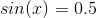, what is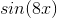ifis betweenand?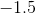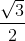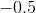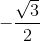Explanation:

Recall that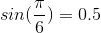.

Therefore, we are looking for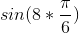or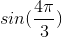.

Now, this has a reference angle of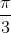, but it is in the third quadrant. This means that the value will be negative. The value of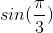is. However, given the quadrant of our angle, it will be.

### Example Question #1 : Sine, Cosine, Tangent

Find the value of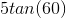in exact form.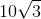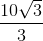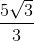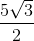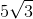Explanation:

Recall that: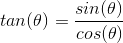This means that: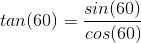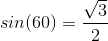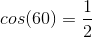Divide the two terms.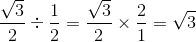This means that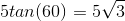.

The answer is: